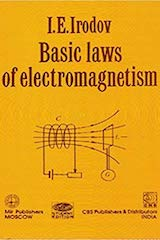# Basic Laws of Electromagnetism by I.E. IrodovApart from Problems in General Physics, IE Irodov also published two related books (1) Fundamental Laws of Mechanics and (2) Basic Laws of Electromagnetism. This article presents a brief review of Basic Laws of Electromagnetism by I.E. Irodov.

This review is limited to the book's utility for IIT JEE. There are 11 chapters in the book. The chapters 3, 7, 8, 10 and 11 have limited utility. We focus on most relevant chapters for JEE, which are

• 1. Electrostatic Field in a Vacuum (leave 1.4)
• 2. A Conductor in an Electrostatic Field (leave 2.5)
• 4. Energy of Electric Field
• 5. Direct Current
• 6. Magnetic Field in a Vacuum
• 9. Electromagnetic Induction
Each chapter begins with a concise theory followed by a number of illustrative examples and solved problems.

## Utility of this Book for IIT JEE

The book contains many standard problems relevant for JEE. The aspirants are advised to go through them. Always keep an eye on JEE syllabus as some problems are irrelevant. These problems are also included in Irodov's popular book Problems in General Physics (with minor changes and without solutions).

The theory part is concise and to the point (terse), as in most of Russian physics books. There is extensive use of vectors and vector calculus. The book excludes all minor details. A few sections are out of JEE syllabus. My advice is to skip it or read it selectively.

Given below is chapter-wise summary:

1. Electrostatic Field in a Vacuum: This chapter contains 10 examples and 11 problems. You may leave section 1.4 on differential form of Gauss's law.
2. A Conductor in an Electrostatic Field: This chapter contains conceptual problems on conductors. You may leave "method of images" given in section 2.5.
3. Electric Field in Dielectrics: In JEE, dielectrics are covered superficially. You may skip this chapter as details like polarization, displacement vectors, boundary conditions are not asked in JEE.
4. Energy of Electric Field: This chapter contains 5 examples and 10 problems.
5. Direct Current: You may leave the continuity equation and generalized Ohm's law (section 5.3). Ten problems given at the end of the chapter are important.
6. Magnetic Field in a Vacuum: It is an important chapter for JEE. Section 6.5 on "differential form of basic laws of magnetic field" may be skipped.
7. Magnetic Field in a Substance: In JEE, this topic is covered superficially. The details given in this chapter are too deep for JEE.
8. Relative Nature of Electric and Magnetic Field: This is beyond JEE syllabus.
9. Electromagnetic Induction: Sections 9.1 to 9.5 are in JEE syllabus. Section 9.6 and 9.7, related to energy in the magnetic field, may be skipped. This is an important chapter for JEE.
10. Maxwell's Equations: This is beyond JEE syllabus.
11. Electric Oscillations: May be skipped as it is beyond JEE syllabus.